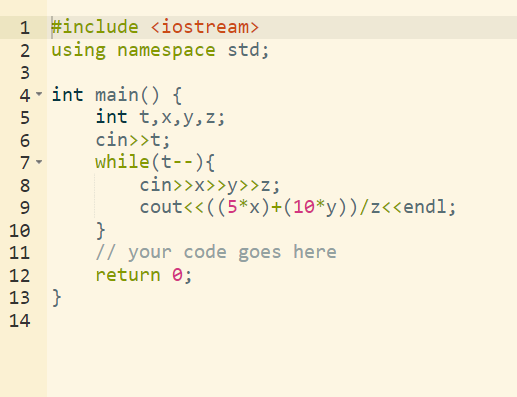# Help me in solving CCHOCOLATES problem

### My issue

include
using namespace std;

void chocolate()
{
int x,y,z;
cin>>x>>y>>x;

cout<<((x5)+(y10));
}

int main() {
int T;
cin>>T;
while(T>0)
{
chocolate();
T–;
}
return 0;
}

I am doing this que by this way, first taking a variable as input for getting the total test cases then a while loop till that input value, and tyen getting into a function named as chocolate() getting values but still not the green signal nfrom here

i have run it on multiple c++ compiler its working there but not here

### My code

``````#include <iostream>
using namespace std;

void chocolate()
{
int x,y,z;
cin>>x>>y>>x;

cout<<((x*5)+(y*10));
}

int main() {
int T;
cin>>T;
while(T>0)
{
chocolate();
T--;
}
return 0;
}
``````

Learning course: Basic Math using C++
Problem Link: CodeChef: Practical coding for everyone

You can do this question in more simple way .You can use if - else instead of make function of chocolate .

1 Like

fine sir I tried again and yes I executed successfully
thank you !

your code was incorrect I have rectified the mistake just have a look what i have changed

``````#include <iostream>
using namespace std;

void chocolate()
{
int x,y,z;
cin>>x>>y>>z;
int total_amount=(x*5)+(y*10);
int ans=total_amount/z;
cout<<ans<<endl;
}

int main() {
int T;
cin>>T;
while(T>0)
{
chocolate();
T--;
}
return 0;
}
``````
1 Like1 Like